## If the boat goes from 30 km/h to 50 km/h over the next 4 h, what is its acceleration? Please help me

Question

If the boat goes from 30 km/h to 50 km/h over the next 4 h, what is its acceleration?

in progress 0
2 months 2021-07-28T01:32:02+00:00 1 Answers 2 views 0

The acceleration is 5km/h

Explanation:

Given

Initial Velocity (u) = 30km/h

Final Velocity (v) = 50km/h

Time (t) = 4h

Required

Determine the acceleration

This question will be answered using the first equation of motion;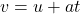Substitute values for u, v and t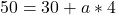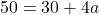Subtract 30 from both sides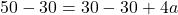Divide both sides by 4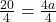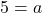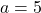Hence;

The acceleration is 5km/h